# Python Program to Convert Hexadecimal to Binary

In this article, we've created some programs in Python, to convert hexadecimal number entered by user to its equivalent binary value. Here are the list of programs:

• Hexadecimal to Binary using while Loop
• Using int() and bin() Methods
• Using user-defined Function
• Using Class

Note - Before creating these programs, if you're not aware about steps used for the conversion, then refer to Hexadecimal to Binary Steps, Formula, Example to get every required things about the topic.

## Hexadecimal to Binary using while Loop

To convert hexadecimal to binary number in Python, you have to ask from user to enter the hexadecimal number, then convert that number into its equivalent binary value as shown in the program given below:

```print("Enter the Hexadecimal Number: ")
hexdecnum = input()

binnum = ""
hexlen = len(hexdecnum)
i = 0
while i<hexlen:
if hexdecnum[i] == '0':
binnum = binnum + "0000"
elif hexdecnum[i] == '1':
binnum = binnum + "0001"
elif hexdecnum[i] == '2':
binnum = binnum + "0010"
elif hexdecnum[i] == '3':
binnum = binnum + "0011"
elif hexdecnum[i] == '4':
binnum = binnum + "0100"
elif hexdecnum[i] == '5':
binnum = binnum + "0101"
elif hexdecnum[i] == '6':
binnum = binnum + "0110"
elif hexdecnum[i] == '7':
binnum = binnum + "0111"
elif hexdecnum[i] == '8':
binnum = binnum + "1000"
elif hexdecnum[i] == '9':
binnum = binnum + "1001"
elif hexdecnum[i] == 'a' or hexdecnum[i] == 'A':
binnum = binnum + "1010"
elif hexdecnum[i] == 'b' or hexdecnum[i] == 'B':
binnum = binnum + "1011"
elif hexdecnum[i] == 'c' or hexdecnum[i] == 'C':
binnum = binnum + "1100"
elif hexdecnum[i] == 'd' or hexdecnum[i] == 'D':
binnum = binnum + "1101"
elif hexdecnum[i] == 'e' or hexdecnum[i] == 'E':
binnum = binnum + "1110"
elif hexdecnum[i] == 'f' or hexdecnum[i] == 'F':
binnum = binnum + "1111"
i = i+1

print("\nEquivalent Binary Value: ", binnum)```

Here is its sample run: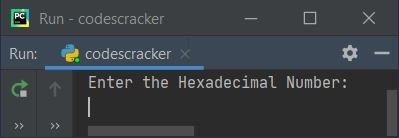Now supply the input say 1D4 as hexadecimal number and press `ENTER` key to convert and print its equivalent binary value as shown in the snapshot given below: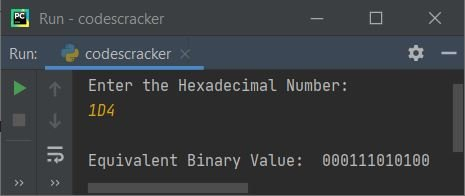The dry run of above program with hexadecimal number input 1D4 goes like:

• Initial values, hexdecnum=1D4 (entered by user), i=0, binnum=""
• The len() method is used to find length of string. Therefore using the following statement:
`hexlen = len(hexdecnum)`
the value 3 (length of 1D4 or hexdecnum) gets initialized to hexlen
• Now the condition (of while loop) i<hexlen or 0<3 evaluates to be true, therefore program flow goes inside the loop
• Inside the loop, I've applied if-elif statements of Python to compare the value stored in hexdecnum one by one to do the task as per current value
• That is, inside the loop, the first condition or condition of if gets evaluated. Since the condition, hexdecnum[i] == '0' or hexdecnum == '0' or '1' == '0' evaluates to be false, therefore program flow does not goes inside this if's body, rather it goes and evaluates the condition of first elif
• That is, the condition hexdecnum[i] == '1' or hexdecnum == '1' or '1' == '1' evaluates to be true, therefore program flow goes inside this elif's body, and binnum + "0001" or "" + "0001" or "0001" gets initialized to binnum
• And the value of i gets incremented by 1. So i=1
• Now program flow goes back and evaluates the condition of while loop again with new value of i
• That is, the condition i<hexlen or 1<3 evaluates to be true again, therefore program flow goes inside the loop again. This process continues until the condition evaluates to be false
• In this way, the value entered in hexadecimal, gets converted into its equivalent binary value using string
• Therefore, after exiting from the loop, just print the value of binnum as binary equivalent of given hexadecimal number by user at run-time

#### Modified Version of Previous Program

This program uses end= to skip printing of an automatic newline using print(). In this program, I've also added an else part to handle the thing when all conditions evaluates to be false.

Inside else, I've assigned 1 to chk and using break keyword, the remaining execution of while loop gets skipped. And after exiting from the loop, either by evaluating its condition as false, or by using break keyword, I've checked the value of chk. That is, if it equal to 0 (initial value), then program flow never goes to else's body. Means, no any invalid hex digit was entered by user. Otherwise print a message like invalid input as shown in the program given below:

```print("Enter the Hexadecimal Number: ", end="")
hnum = input()

bnum = ""
chk = 0
hlen = len(hnum)
i = 0
while i<hlen:
if hnum[i] == '0':
bnum += "0000"
elif hnum[i] == '1':
bnum += "0001"
elif hnum[i] == '2':
bnum += "0010"
elif hnum[i] == '3':
bnum += "0011"
elif hnum[i] == '4':
bnum += "0100"
elif hnum[i] == '5':
bnum += "0101"
elif hnum[i] == '6':
bnum += "0110"
elif hnum[i] == '7':
bnum += "0111"
elif hnum[i] == '8':
bnum += "1000"
elif hnum[i] == '9':
bnum += "1001"
elif hnum[i] == 'a' or hnum[i] == 'A':
bnum += "1010"
elif hnum[i] == 'b' or hnum[i] == 'B':
bnum += "1011"
elif hnum[i] == 'c' or hnum[i] == 'C':
bnum += "1100"
elif hnum[i] == 'd' or hnum[i] == 'D':
bnum += "1101"
elif hnum[i] == 'e' or hnum[i] == 'E':
bnum += "1110"
elif hnum[i] == 'f' or hnum[i] == 'F':
bnum += "1111"
else:
chk = 1
break
i = i+1

if chk==0:
print("\nEquivalent Binary Value = ", bnum)
else:
print("\nInvalid Input!")```

Here is its sample run with hexadecimal input 24YD: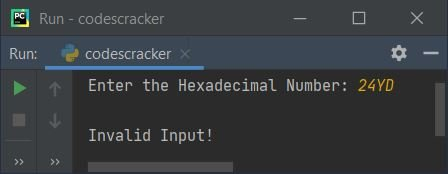Note - Since Y is not a valid hex digit, therefore the above output, Invalid Input was produced.

## Hexadecimal to Binary using int() and bin()

This program uses int() and bin() methods to do the same job in more precised code as Python known for.

```print("Enter the Hexadecimal Number: ", end="")
hnum = input()

hnum = int(hnum, 16)
bnum = bin(hnum)
print("\nEquivalent Binary Value = ", bnum)```

Here is its sample run with user input, 1DF3 as hexadecimal number input: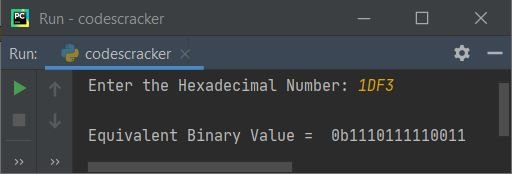Note - To print only binary value, that is to skip first two characters from the above output, put [2:] just after the bnum variable while printing. That is, replace the following statement:

`print("\nEquivalent Binary Value = ", bnum)`

with the statement given below:

`print("\nEquivalent Binary Value = ", bnum[2:])`

Now the output looks like: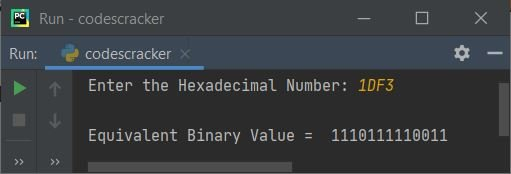## Hexadecimal to Binary using Function

This program is created using user-defined and predefined functions. The name of user-defined function used in this program is HexToBin(). This function receives a value as its argument and returns its equivalent binary value using bin() and int() method.

```def HexToBin(h):
return bin(int(h, 16))

print("Enter the Hexadecimal Number: ", end="")
hnum = input()

bnum = HexToBin(hnum)
print("\nEquivalent Binary Value = ", bnum[2:])```

## Hexadecimal to Binary using Class

This is the last program of this article, created using a class named CodesCracker, an object-oriented feature of Python.

```class CodesCracker:
def HexToBin(self, h):
return bin(int(h, 16))

print("Enter the Hexadecimal Number: ", end="")
hnum = input()

obj = CodesCracker()
bnum = obj.HexToBin(hnum)
print("\nEquivalent Binary Value = ", bnum[2:])```

In this program, an object named obj of class CodesCracker is created to access its member function named HexToBin() using dot (.) operator. Rest of the things works like a normal function.

#### Same Program in Other Languages

Python Online Test

« Previous Program Next Program »